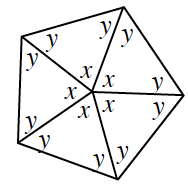### Home > INT2 > Chapter 8 > Lesson 8.2.1 > Problem8-26

8-26.

Examine the geometric relationships in the diagram below. Show all of the steps in your solutions for $x$ and $y$. Name the polygon.

$x + x + x + x + x = 360^\circ$

$2y + x = 180^\circ$